Function Repository Resource:

# StrongPrimeQ

Determine whether a number is a strong prime

Contributed by: Arnoud Buzing
 ResourceFunction["StrongPrimeQ"][p] gives True if p is a strong prime and False otherwise.

## Details and Options

ResourceFunction["StrongPrimeQ"] uses the number theoretic definition of a strong prime, not the cryptographic one.
A prime is strong if it is greater than the arithmetic average of its nearest primes above and below.

## Examples

### Basic Examples (4)

The prime number 457 is strong:

 In:=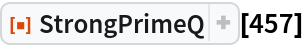Out=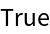To show this, we must show that 457 is bigger than the average of its preceding and succeeding primes. First, find the number of primes less than or equal to 457:

 In:=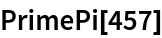Out=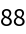Because 457 is the 88th prime, we consider the 87th and 89th primes:

 In:=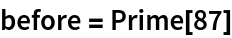Out=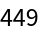In:=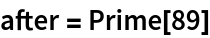Out=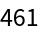Now, test that 457 is bigger than the average of these:

 In:=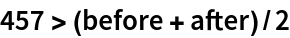Out=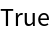Arnoud Buzing

## Version History

• 1.0.0 – 29 April 2020